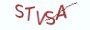# 3.3.1 算法思路

(1) 14除以8，商为1，余数为6

(2) 8除以6，  商为1，余数为2

(3) 6除以2，  商为3，余数为0

# 3.3.2 定理证明

k 为 n 除以 p 的商，r 为 n 除以 p 的余数。

# 3.3.3 算法实现

```# __author__ = 薯条老师

import random

def gcd(a, b):
while a != 0:
a, b = b % a, a
return b

if  __name__ == "__main__":
a, b = random.randint(1, 1000), random.randint(1,  1000)
print("{}与{}的最大公约数:{}".format(a,b, gcd(a,  b)))```

```# __author__ = 薯条老师

import random

def gcd(a, b):
return b if a == 0 else gcd(b % a, a)

if __name__ == "__main__":
a, b =  random.randint(1, 1000), random.randint(1,  1000)
print("{}与{}的最大公约数:{}".format(a, b,  gcd(a, b)))```

# 3.3.4 最具实力的小班培训

`(1) Python后端工程师高薪就业班，月薪11K-18K，免费领取课程大纲(2) Python爬虫工程师高薪就业班，年薪十五万，免费领取课程大纲(3) Java后端开发工程师高薪就业班，月薪11K-20K, 免费领取课程大纲(4) Python大数据分析，量化投资就业班，月薪12K-25K,免费领取课程大纲`## 注册账号